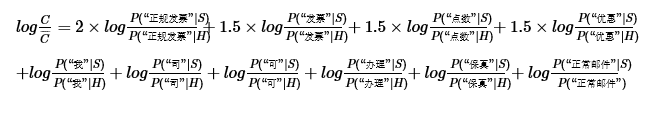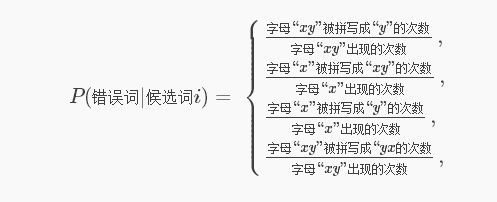# 朴素贝叶斯(二)

1. 简单高效，吊丝逆袭

“有人对此提出了一个理论解释，并且建立了什么时候朴素贝叶斯的效果能够等价于非朴素贝叶斯的充要条件，这个解释的核心就是：有些独立假设在各个分类之间的分布都是均匀的所以对于似然的相对大小不产生影响；即便不是如此，也有很大的可能性各个独立假设所产生的消极影响或积极影响互相抵消，最终导致结果受到的影响不大。具体的数学公式请参考这篇 paper。”（刘未鹏《：平凡而又神奇的贝叶斯方法》）

9. 处理重复词语的三种方式

“代开发票。增值税发票，正规发票。”

（“代开”,“发票”,“增值税”,“发票”,“正规”,“发票”）

9.1 多项式模型：

P(（“代开”,“发票”,“增值税”,“发票”,“正规”,“发票”)|S）
=P(“代开””|S)P(“发票”|S)P(“增值税”|S)P(“发票”|S)P(“正规”|S)P(“发票”|S）
=P(“代开””|S)P3(“发票”|S)P(“增值税”|S)P(“正规”|S)

P(“发票”|S）=每封垃圾邮件中出现“发票”的次数的总和/每封垃圾邮件中所有词出现次数（计算重复次数）的总和

9.2 伯努利模型

P(（“代开”,“发票”,“增值税”,“发票”,“正规”,“发票”)|S）
=P(“发票”|S)P(“代开””|S)P(“增值税”|S)P(“正规”|S）

P(“发票”|S）=出现“发票”的垃圾邮件的封数/每封垃圾邮件中所有词出现次数（出现了只计算一次）的总和

9.3 混合模型10. 去除停用词与选择关键词

“停用词”和“关键词”一般都可以提前靠人工经验指定。不同的“停用词”和“关键词”训练出来的分类器的效果也会有些差异
11. 浅谈平滑技术

P(（“我”,“司”,“可”,“办理”,“正规发票”)|S)
=P(“我”|S)P(“司”|S)P(“可”|S)P(“办理”|S)P(“正规发票”|S）

P(“正规发票”|S）=(出现“正规发票”的垃圾邮件的封数+1)/(每封垃圾邮件中所有词出现次数（出现了只计算一次）的总和+2)

P(“发票”|S）=(每封垃圾邮件中出现“发票”的次数的总和+1)/(每封垃圾邮件中所有词出现次数（计算重复次数）的总和+被统计的词表的词语数量)

1. 小结

13. 为什么不直接匹配关键词来识别垃圾邮件？

13.工程上的一些tricks

13.1 trick1：取对数

C=P(“我”|S)P(“司”|S)P(“可”|S)P(“办理”|S)P(“正规发票”|S)
×P(“保真”|S)P(“增值税”|S)P(“发票”|S)P(“点数”|S)P(“优惠”|S)P(“垃圾邮件”)
C¯¯¯=P(“我”|H)P(“司”|H)P(“可”|H)P(“办理”|H)P(“正规发票”|H)
×P(“保真”|H)P(“增值税”|H)P(“发票”|H)P(“点数”|H)P(“优惠”|H)P(“正常邮件”)

logC=logP(“我”|S)+logP(“司”|S)+logP(“可”|S)+logP(“办理”|S)+logP(“正规发票”|S)
+logP(“保真”|S)+logP(“增值税”|S)+logP(“发票”|S)+logP(“点数”|S)+logP(“优惠”|S)+logP(“垃圾邮件”)
logC¯¯¯=logP(“我”|H)+logP(“司”|H)+logP(“可”|H)+logP(“办理”|H)+logP(“正规发票”|H)
+logP(“保真”|H)+logP(“增值税”|H)+logP(“发票”|H)+logP(“点数”|H)+logP(“优惠”|H)
“做对数运算岂不会也很花时间？”的确如此，但是可以在训练阶段直接计算 logP ，然后把他们存在一张大的hash表里。在判断的时候直接提取hash表中已经计算好的对数概率，然后相加即可。这样使得判断所需要的计算时间被转移到了训练阶段，实时运行的时候速度就比之前快得多，这可不止几个数量级的提升。
13.2 trick2：转换为权重

logC/C¯=logP(“我”|S)/P(“我”|H)+logP(“司”|S)/P(“司”|H)+logP(“可”|S)/P(“可”|H)+logP(“办理”|S)/P(“办理”|H)+logP(“正规发票”|S)/P(“正规发票”|H)
+logP(“保真”|S)/P(“保真”|H)+logP(“增值税”|S)/P(“增值税”|H)+logP(“发票”|S)/P(“发票”|H)+logP(“点数”|S)/P(“点数”|H)+logP(“优惠”|S)/P(“优惠”|H)+logP(“正常邮件”|S)/P(“正常邮件”)
logC/C¯ 如果大于0则属于垃圾邮件。我们可以把其中每一项作为其对应词语的权重，比如logP(“发票”|S)/P(“发票”|H) 就可以作为词语“发票”的权重，权重越大就越说明“发票”更可能是与“垃圾邮件”相关的特征。这样可以根据权重的大小来评估和筛选显著的特征，比如关键词。而这些权重值可以直接提前计算好而存在hash表中 。判断的时候直接将权重求和即可。13.3 trick3：选取topk的关键词13.4 trick4：分割样本

13.5 trick5：位置权重13.6 trick6：蜜罐

14. 贝叶斯方法的思维方式

P(Y|X)=P(X|Y)/P(Y)P(X)

4.2 处理多分类问题

P(Y1|X)=P(X|Y1)P(Y1)/P(X)
P(Y2|X)=P(X|Y2)P(Y2)/P(X)
P(Y3|X)=P(X|Y3)P(Y3)/P(X)

P(Yi|X)∝P(X|Yi)P(Yi)；i=1,2,3

4.3 先验概率的问题

P(Yi|X)∝P(X|Yi)；i=1,2,3

P(Y1)=5000/25000=1/5，

P(Y2)=P(Y3)=10000/25000=2/5

15. (朴素)贝叶斯方法的常见应用

15.1 褒贬分析

15.2 拼写纠错

P(候选词i|错误词)∝P(错误词|候选词i)P(候选词i)；i=1,2,3,…

P(候选词i)=候选词出现的次数/所有词出现的次数

P(错误词|候选词i)=候选词被拼写成该“错误词”的次数/候选词出现的次数16. 小结

08-1401-23
04-24
04-0994
02-014万+
11-07
05-12
12-02
12-1668
12-23451
03-183844
01-035234
06-02
©️2020 CSDN 皮肤主题: 博客之星2020 设计师:CY__